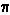D

#### What is pi?

3 viewed last edited 3 years agoRejuvenatedAfricanBuffalo
0Sangeetha Pulapaka
0

Pi is a name given to the ratio of the circumference of a circle to the diameter. That means, for any circle, you can divide the circumference (the distance around the circle) by the diameter and always get exactly the same number. It doesn't matter how big or small the circle is, Pi remains the same. Pi is often written using the symboland is pronounced "pie", just like the desserJokes aside, the value of pi in mathematics is approximately equal to 3.14. Because Pi is known to be an irrational number it means that the digits never end or repeat in any known way. But calculating the digits of Pi has proven to be an fascination for mathematicians throughout history. Some spent their lives calculating the digits of Pi, but until computers, less than 1,000 digits had been calculated. In 1949, a computer calculated 2,000 digits and the race was on. Millions of digits have been calculated, with the record held (as of September 1999) by a supercomputer at the University of Tokyo that calculated 206,158,430,000 digits. (first 1,000 digits)